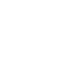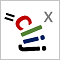# CLIJ2GPU accelerated image processing for everyone

CLIJ2 home

## generateLabelFeatureImageGenerates a feature image for Trainable Weka Segmentation.

Use this terminology to specify which features should be generated:

• BOUNDING_BOX_DEPTH
• BOUNDING_BOX_WIDTH
• BOUNDING_BOX_HEIGHT
• CENTROID_X
• CENTROID_Y
• CENTROID_Z
• MASS_CENTER_X
• MASS_CENTER_Y
• MASS_CENTER_Z
• MAX_DISTANCE_TO_CENTROID
• MAX_DISTANCE_TO_MASS_CENTER
• MEAN_DISTANCE_TO_CENTROID
• MEAN_DISTANCE_TO_MASS_CENTER
• MAX_MEAN_DISTANCE_TO_CENTROID_RATIO
• MAX_MEAN_DISTANCE_TO_MASS_CENTER_RATIO
• MAXIMUM_INTENSITY
• MEAN_INTENSITY
• MINIMUM_INTENSITY
• SUM_INTENSITY
• STANDARD_DEVIATION_INTENSITY
• PIXEL_COUNT
• local_mean_average_distance_of_touching_neighbors
• local_maximum_average_distance_of_touching_neighbors
• count_touching_neighbors
• local_minimum_average_distance_of_touching_neighbors
• average_touch_pixel_count
• local_minimum_count_touching_neighbors
• average_distance_n_closest_neighbors
• average_distance_of_touching_neighbors
• local_mean_count_touching_neighbors
• local_mean_average_distance_n_closest_neighbors
• local_maximum_average_distance_n_closest_neighbors
• local_standard_deviation_average_distance_of_touching_neighbors
• local_maximum_count_touching_neighbors
• local_standard_deviation_count_touching_neighbors
• local_standard_deviation_average_distance_n_closest_neighbors
• local_minimum_average_distance_n_closest_neighbors

Example: “MEAN_INTENSITY count_touching_neighbors”

### Usage in ImageJ macro

``````Ext.CLIJx_generateLabelFeatureImage(Image input, Image label_map, Image label_feature_image_destination, String feature_definitions);
``````

Imprint